A New Methodology to Solve Joint Energy and Primary Reserve Scheduling | OMICS International
Global Journal of Technology and Optimization

# A New Methodology to Solve Joint Energy and Primary Reserve Scheduling

1Electrical Engineering Department, Shahid Bahonar University of Kerman, Iran

2South Kerman Electrical Distribution Company

*Corresponding Author:
M. Rouholamini
Electrical Engineering Department
Shahid Bahonar University of Kerman, Iran
E-mail: [email protected], [email protected], [email protected]

Received date: February 2012, Revised date: May 2012, Accepted date: June 2012

Visit for more related articles at Global Journal of Technology and Optimization

#### Abstract

The unit commitment problem has been broadly studied in recent years in many researches. However, this is not all of the important tasks for independent system operator. One of the most important tasks is the optimal provision of primary frequency regulation reserve with the lowest cost that should be considered in the unit commitment problem. Frequency control in power system, as an ancillary service, has closed dependence to hourly scheduling of energy. In this paper, a novel approach is proposed to solve simultaneous scheduling of energy and primary reserve using genetic algorithm. The proposed methodology is fast and simple against conventional methods. In addition, applicability of scheduled primary reserve has been considered in optimization process. Finally, simulation results for a 17 unit case study are presented in comparison with a related work. The simulation results using Matlab 2009a are presented which verify the effectiveness and robustness of the proposed method.

#### Introduction

Fast growing load in power systems associated with a large gap between heavy load and light load periods, generation scheduling and unit commitment (UC, hereinafter) has become a crucial issue in operation time horizon and UC problem has always been an important research challenge in power systems and especially under restructured environment. On the other hand, sudden variations or unwanted changes in system demand may cause a frequency deviation in power systems. Frequency control is one of the most important tasks of independent system operator (ISO, hereinafter). In restructured electricity industry. In power systems, frequency control can be considered as an ancillary service that will be provided using the eligible resources made available by market participants which make UC problem more complicated. Usually, generation reserve capability is called as "frequency control reserve", which is classified as primary, secondary and tertiary reserve depends on their response time and how they are deployed [1, 2]. Primary reserve is provided by generating units through their local droop characteristic in response to system frequency deviation from nominal, a closed-loop process denoted here as primary frequency regulation. This is the fastest of the three reserve control strategies with a response time of the order of seconds. The secondary reserve, which has a response time of the order of minutes, known as automatic generation control (AGC) and more specifically Load Frequency Control (LFC), is applied to regulate the area control-error (ACE) . ACE regulating is compensated via tertiary reserve. It should be noted that, for regulating ACE, all operational constraints must be satisfied . The tertiary reserve, with a response time of the order of minutes, can be used for congestion management, improvement of lost reserves and compensation of the incomplete tasks that has not done by AGC. In this paper, primary reserve scheduling is emphasized while secondary and tertiary reserve has not been considered.

In addition, provision of primary frequency services is functional to hourly scheduling of energy [5, 6]. The used value for primary reserve of each unit depends on the frequency deviation occurred due to the lost generating units. Furthermore, scheduling of energy and ancillary services (AS) are developed using simultaneously or sequentially methods in different countries. Form market point of view, energy and ancillary services are transacted simultaneously while technically ancillary services will be produced after preparation of energy in sequential scheduling. AS must be sorted qualitatively in sequential scheduling second method, in other words the primary reserves are scheduled firstly while the secondary & tertiary reserves will be scheduled hierarchically. In ancillary service market the reserve with lower quality can be replaced by the reserve with higher quality. It means that the primary reserve can be used for secondary reserve, if it is necessary. Using sequential scheduling method, it is possible that "price reversal" be occurred, where this event has been experienced in California and Newengland . Simultaneous scheduling is more complex in comparison with sequential scheduling but the global optimal solution is accessible in Simultaneous method. In fact, in sequential scheduling, the outputs of generating units are input data as some constant parameters which are obtained from UC. So this method cannot warrant the achievement of globally optimal solutions. In addition, it is possible, the final solution of sequential method be not practical. However, as scheduling of energy and reserves are strongly coupled, scheduling them simultaneously will be more advantageous [5, 6] ending with a higher social welfare .

The work reported in  is one of the most important researches about scheduling of energy and primary reserves which has used an iterative economic dispatch. The values of generation and reserve of each unit are modified successively in aforementioned paper. In , operational and stability constraints have been considered in scheduling problem using decision tree solution method. However, in both of the above mentioned approaches, generation is scheduled a priori and then the reserve is scheduled, in the other words this is the sane sequentially scheduling. Restrepo et al proposed an approach in formulation of unit commitment considering primary and tertiary reserve constraints simultaneously in ; they have used GAMS software to solve the simultaneous scheduling as a mixed integer linear problem using linearization of quadratic cost function. The paper published by Galiana and colleagues indicates that simultaneous method increases social welfare . In , Rajabi mashhadi et al have published a paper about impacts of capabilities and constraints of generating units on simultaneous scheduling of energy and primary reserve but all of the proposed constraints in  is not considered in this paper. , ,  are other papers which are associable to provision of frequency reserves.

In the work reported by Ebrahimi and Mozafari, optimal load frequency control in a two-area interconnected power system based on genetic algorithm is presented .

This seems that the scheduling of energy and primary reserve is studied in several researches using simultaneous and sequential methods. However, the mathematical configuration of simultaneous scheduling of energy and reserve has not been attended by authors, in the other words they have focused on final solution instead of solution method.

This paper is focused on simultaneous scheduling of energy and primary reserve. The reserve should suffice for compensating large and sudden outages, in the form of loss of one or more generating units, in an isolated power system.

In this paper, the proposed method breaks the original simultaneous scheduling problem in two optimization problem including an inner and an outer loop. But this is not a sequential technique.

To obtain the globally optimal scheduling of energy and reserve, a heuristic iterative method based on genetic algorithm will be used in outer loop and quadratic programming technique is used to solve the non linear quadratic problem in inner loop. All operational and general constraints for scheduling of energy and reserve presented in previous works are considered in proposed formulation. The simulation results verify the accuracy and rapidity of the proposed methodology in comparison with previous methods. In addition, the final solution is applicable and the method needs low computational time.

Problem formulation

In this section, problem formulation of simultaneous scheduling of energy and primary reserve for an isolated power system are presented. It should be noted that loads respond to frequency deviation inherently, denoted as load damping effect, however it is neglected in following problem formulation. The power market in this paper is assumed as pay as bid and the contingency has been considered as N-m though the contingency is considered in the form of N-1 in simulation results.

According to previous researches the objective function to minimize can be expressed as Eq(1), where the objective function includes operation cost as first part and primary reserve cost as second part.

It should be noted that primary reserve scheduling costs consist of two terms, 1) Preparation costs and 2) deployment costs. In continue, the second part is neglect in problem formulation and just the first part of these costs is considered and just primary reserve is discussed in problem formulation. Full considering for primary, secondary and tertiary reserve is given at appendix.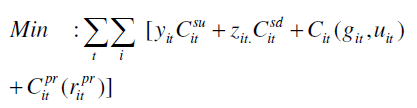(1)

Operation costs include start-up, shut-down and running costs. It is assumed that running costs of generating units are defined by a quadratic polynomial as equation (2) and primary reserve is assumed as a simple linear equation as Eq(3), ,,.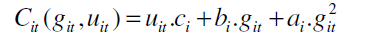(2)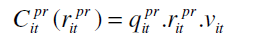(3)

In continue, the general constraints of simultaneous scheduling are presented.

I) Pre-contingency system power balances, here the system loss has been neglected.

In condition of considering power losses, total generating output must be equal to demand plus loss during each hour.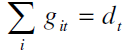(4)

II) Specified limits of generating units as below.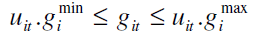(5)

III) Upper limit of primary reserve, in this paper, contingencies defined by loss of pre-specified combinations of generating units. This implies that following each contingency, only negative frequency deviations will occur. Figure 1 illustrates the relation between primary reserve and frequency deviation for an arbitrary unit i. the upper generation bound,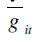,used in Eq(6) is the maximum output of unit i under primary frequency regulation, defined by either the unit frequency-regulation ramp limit,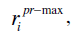or by unit generating capacity limit,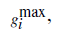whichever is smaller. Unit frequency-regulation ramp limit is the maximum reserve that a unit can produce within 10 seconds following a contingency.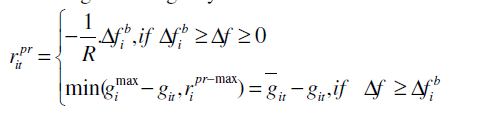(6)

On the other hand, the relation between primary reserve and frequency deviation is linear according to droop of unit before the vertical line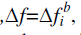and for frequency deviation more than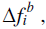it is restricted to unit generating capacity limit or ramp up limit.

IV) summation of scheduled primary reserves of participating units must be greater than or equal to total lost generation, Since generation and demand must balance under each of the order of contingency, the remaining healthy generating units must provide enough reserve to make up for the lost generation under any contingency, occurring during any time interval t of the scheduling horizon. These requirements for primary reserve are as below: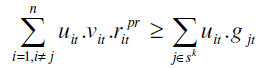(7)

The above inequality restricts the values of primary reserves to a lower limit.

V) Critical system frequency deviation allowed, to avoid load shedding by under-frequency relays, the frequency deviations must be limited as follows: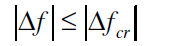(8)

Where,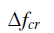is the minimum allowed negative frequency deviation. This constraint represents that the employment of primary reserve should not be entailed to an inordinate decline in nominal frequency. Athwart the aspect of relation (8) seems to be a nonlinear constraint but it is a linear constraint unlike what it seems to be. so it is possible that the above relation be rewritten as below: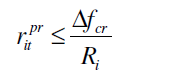(9)

It should be mentioned that both of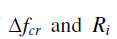are per unit parameters. Since we defined two upper restrictions in addition to Eq(9) heretofore so a general relation is able to be written as follows: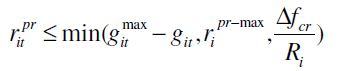(10)

VI) The scheduled primary regulation reserve for unit i,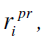must be greater than or equal to the maximum generation deviation relative to the pre- contingency level over all contingencies .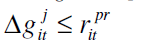(11)

The final solution of scheduling problem will not be applicable if the above constraint is not satisfied. This important matter is not considered in  during optimization process and explained in  perfectly. This is the single nonlinear constraint of simultaneous scheduling of energy and primary reserve which is considered in solution method using a novel approach in this paper.

Other conventional constraints for short time scheduling of unit commitment such as minimum up time, minimum down time, start up cost and shut down cost of units can be considered in optimization process. However, in this paper, only start up cost constraint has been considered.

Proposed solution method

The type of simultaneous scheduling of energy and primary reserve is a Mixed Integer Non Linear Programming (MINLP) problem . Where the binary variables are the representation of on/off status and continual variables represent the values of generation and reserves. In solving this problem two important challenges arise: 1)the fact that primary reserve of unit i must lie on the piece-wise curve imposed by Eq(6), particularly considering that the elbow of this curve is described by two decision variables, one is the saturation level ,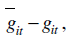and the corresponding frequency deviation is another. 2) The fact according to Eq (12), the variable,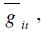is defined as the minimum of two variables .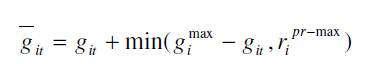(12)

The performance of genetic algorithm does not depend on derivable or convexity of the objective function and here the optimization problem is a MILNP problem. The genetic algorithm which is one of most popular heuristic methods is capable to converge to global optimal solution . More about Genetic Algorithm are expressed at the appendix. Genetic algorithm has been commonly applied to solve similar optimization problems , . In this method, the ineligible statuses of units have been sieved in optimization process using some algebraic analysis on the mathematical structure of simultaneous scheduling problem. Although the proposed method can be used in a multi period scheduling but the method only is implemented on a single period scheduling for simplicity. The primary process of solution method has been extracted from  but considering all of the proposed constraints in  for simultaneous scheduling problem.

In continue it is assumed the AGC is not installed on the system and tertiary reserve will be prepared subsequently so the secondary and tertiary reserves are not considered.

Encoding binary variables

In this paper, it is assumed that each unit can lie in three modes which are: 1) unit is shut down, 2) unit is turn on but does not participate in frequency control, 3) unit is turn on and has participated in frequency control as shown in table 1

According to the above table, the status of each unit can be defined by two bits with a decimal upper limit restricted to three . Figure 2 illustrates a schematic representation of an individual chromosome typically.

Determination of solution feasibility

In , several beneficial relations are presented to identify and eliminate the infeasible chromosomes from candidate solutions in genetic algorithm. Some of them are the same preconditions of unit commitment problem and some are extracted based on specified limitations including ram up limit and generation limits.

In order to express the proposed method we recast the Eq (10) as below: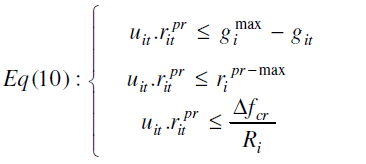(13)

The expression of Eq (15) is indicated in Figure 3. Figure 3 illustrates that three bounds are defined for primary reserve. There are twins on the vertical axis due to spinning capacity limit and ramp up limit and one on the horizontal axis due to critical frequency deviation allowed.

Now assume a contingency of the order of N-1 has occurred, in the other words, system operator has lost one of generating units and system frequency is fallen at a final value in steady state. Draw a virtual vertical line at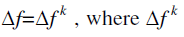is the steady stat frequency deviation. Obviously the virtual line hits the horizontal bounds and slopping line. So we have three reserves for each participating unit but the minimum value is correct according to (10). It is clearly that the system frequency reaches steady-state at a value that causes the sum of the on-line generators output to be equal to the system load so the sum of the reached primary reserves must be equal to lost generation in steady state formed as equation (14).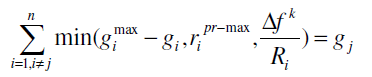(14)

Since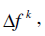is less than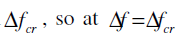the relation (14) can be recast as (15).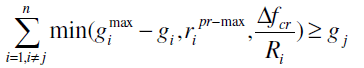(15)

The left part of the above relation is a sum of minimum values of several terms so it can be rewritten as below from mathematical point of view.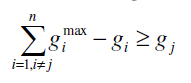(16)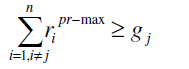(17)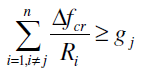(18)

Notice that many relations in addition to the above equations can be written; for the same reason the above relations are some necessary conditions and not enough conditions. If we write the Eq (16) for all sets of lost generating units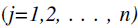and then sum them to each other, with attention to equation (4), we have: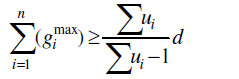(19)

similarly, if the above computation be implemented on the relation (17) and (18) , new preconditions will be obtained as follows: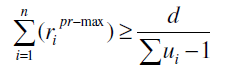(20)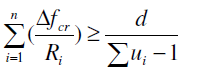(21)

Relations (19- 21) are valid against contingency of the order of N-1. For condition of contingency with order of N-m the relations (22-25) can be used to eliminate the impossible status of units.

These preconditions assure that binary genetic algorithm works with feasible chromosomes.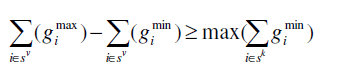(22)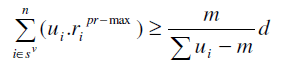(23)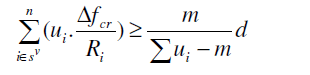(24)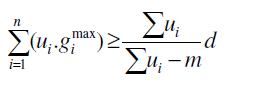(25)

Figure 4 Shows the flowchart of the proposed solution method based on application of defined preconditions. The flowchart shows the general flow for the various stages of our method. While initial population, in the method, is randomly generated, binary variables are encoded especially. in each step, population is checked for the solution feasibility and infeasible strings are eliminated. Therefore, new random populations are generated. This would ensure that only feasible strings are considered for solving the problem of simultaneous scheduling of energy and primary reserve. Major steps in flowchart are explained as below:

In first stage, the problem data are collected. In the second step, a set of initial values is generated randomly for binary variables. Third step according to proposed preconditions determines the feasibility of binary population. In fourth step, the values of primary reserves and generation outputs, for feasible binary variables, will be obtained solving the linear constrained quadratic program. In this step also the fitness function for feasible subset of binary population will be computed. The fitness function will be penalized for infeasible subset of binary population. In fifth step the values of fitness function with binary population are sent to genetic algorithm. New population generated by genetic algorithm is replaced by old population and this process, steps 1 to 5, will be continued until the stopping criterion is satisfied. In sixth step, the optimal solution will be applied to a modificative algorithm.

In the flowchart, Blok 4 and 18 are not embedded in previous work reported in . Here, Blok 4 prevents ineligible strings and Blok 18 reduces computational time because it is done outside of optimization process. Blok 18 tasks to justify the constraint defined by Eq (11). In the other words, in Blok 17 optimal generations and primary reserves are defined but it is possible that the post contingency generation deviation of healthy generating units be greater than scheduled primary reserves for some generating units . So the scheduled primary reserves must be modified finally, this important is the task of Blok 18.

Figure 5 illustrates the modificative algorithm for a typical 4 unit case study.

After determination of generations and reserves, Figure 5 can be depicted and then the vertical line,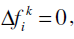can be drawn while one(or more) of units has been lost. This line hit the steady state speed characteristic (droop) of participating units. Then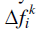will be increased continually while total sum of reserves to be equal to lost generation. This method is employed for other units successively and table 2 will be obtained finally.

Amounts needed for primary reserves are the maximum values of any column of table 2 for each participating unit.

Although in this paper the regulated primary reserves are being reapproved but this is different in comparison with sequential scheduling and not be mistaken with sequential method because in sequential method the generating units output (gi) are obtained from unit commitment and economic dispatch.

Case study and simulation results

In this section the proposed method to solve simultaneous scheduling of energy and primary reserve is tested on an isolated power system including 17-generating units. This system is scheduled over a 1-h horizon, using generator data from an existing study . System frequency and maximum allowed frequency deviation have been assumed to be 50 Hz and -500mhz, respectively. The demand of the system has been to be 1500MW. The droops of all units is assumed to be 5%. In this case, loss of one generating unit (N-1) at a time has been considered as the security criterion ,. The initial population, in the method, is randomly generated; population size and the maximum generation of GA are 20 and 200, respectively. Crossover and mutation probabilities are considered to be 0.8 and 0.01, respectively.

Table 3 presents the number of infeasible status in comparison with all of possible status of units which are identified using proposed preconditions in (19-25). The feasible search space of optimization problem is around 15% of the initial search space. Initial search space includes ineligible and eligible status. Whereas it was around 87% of initial search space if the proposed preconditions be not used. So there is a vast search space reduction for a highly constrained mixed integer optimization problem.

Furthermore, the feasible search space is able to be reduced more using rather extractable relations, but inordinate reduction in feasible search space hazards the genetic algorithm convergence because we are generating initial population randomly. In other words, there is a tradeoff between convergence time and initial feasibility.

In Table 4, generation output and primary reserve using proposed method are presented in comparison with results in , for the same case study.

The results express that the operation costs are declined 7% compared to . Furthermore the computational time improvement is the additional advantage of this methodology. Figure 6 illustrate the primary reserve levels and break frequency for each participating unit. This is a graphical expression of table 4.

Relation (8) presents the allowed frequency deviation limit. According to the first assumpsit the frequency deviation must be less than or equal to 500 mhz following loss of any generating unit. If we shut down an individual generating unit due to a virtual contingency in the form of loss of one generating unit, Δf k and desired primary reserve values will be obtained for all generating units as shown in Table 5 and Table 6, respectively. Table 5 indicates that the maximum frequency deviation is equal to 324.4 MHz against outage of A, B, E, F, J. frequency deviation will be at minimum value following loss of unit P because generation output for this unit is smallest output value (Table 4). Whereas, the relation (11) is considered in problem formulation, so the desired primary reserves (Table 6) , altogether, are less than scheduled primary reserves unlike the previous works such as .

Table 5: The values of steady state frequency deviation following loss of any generating unit

It should be mentioned that maximum Δf ki is less than or equal to maximum break frequency deviation (Δf i b). Here, it is satisfied as 324.4<324.7.

Some of frequency deviations in Table 6 are equal to zero because some of units don’t participate in frequency control.

Presented numbers in Table 4 as scheduled primary reserves are the maximum values of the respective column of Table 6.

Figure 7 shows the convergence trend of the genetic algorithm during 200 generations. Since the ineligible chromosomes are penalizing, hence they have much expense and been appeared just in first generations.

#### Conclusion

A 1-hour unit commitment problem subject to primary regulation reserve constraints is formulated in this paper. This problem has not received as much attention in the previous works. It is considered that some participating generating units are allowed to do not participate in primary frequency control due to defining binary variable vi in problem formulation. Since the type of simultaneous Scheduling problem is MINLP, so a novel method based on binary genetic algorithm is proposed. In this research, only credible contingencies, negative frequency deviation following each contingency have been considered.

In this novel method, statuses of generating units are expressed as binary variables. In addition, some preconditions are presented to identify the ineligible chromosomes in each population which have not also received enough attention too. These preconditions very seriously will cause a significant reduction in the computational time.

Finally, the proposed approach has been implemented on an isolated power system and the simulation results are presented in comparison with a previous work. Results indicate that the final solution is practical and more optimal. The proposed method is easy to implement with low computational time and numeric results show that our method has the following merits: efficient searching ability, robustness in result.

#### Acknowledgment

Many thanks go in particular to Mr.Ghasemnejad for his contributions during the preparation of this paper.

#### References

Select your language of interest to view the total content in your interested language

### Article Usage

• Total views: 11852
• [From(publication date):
December-2012 - Dec 09, 2019]
• Breakdown by view type
• HTML page views : 8078Can't read the image? click here to refresh